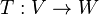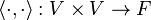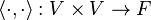# Stupid Question about a Notation in Linear algebra

• I
I know the arrow -> means a map.

For example,defines a linear map. But I cannot figure out what does a X mean?I know that above denote a inner product map. whatever is on the left of the : is defined by whatever is on the right. but what is the x symbol? What is the correct way to read it? Is there a basic book that can tell me more about these symbols?

fresh_42
Mentor
I know the arrow -> means a map.

For example,defines a linear map. But I cannot figure out what does a X mean?I know that above denote a inner product map. whatever is on the left of the : is defined by whatever is on the right. but what is the x symbol? What is the correct way to read it? Is there a basic book that can tell me more about these symbols?
It describes the set of all pairs ##(u,v)## with elements ##u,v \in V##. It is called cartesian product.
https://en.wikipedia.org/wiki/Cartesian_product

•smodak
I guess it denotes a bil
It describes the set of all pairs ##(u,v)## with elements ##u,v \in V##. It is called cartesian product.
https://en.wikipedia.org/wiki/Cartesian_product

Ah-Ha! I am a software engineer. I know what a cartesian product is (use it all the time in SQL). I had no idea that's what it meant :) Thanks a lot.

Which branch of math is this? I know basic linear algebra and basic set theory but never used such symbols as I showed above. Is there any suitable book for this?

fresh_42
Mentor
I guess it denotes a bil

Ah-Ha! I am a software engineer. I know what a cartesian product is (use it all the time in SQL). I had no idea that's what it meant :) Thanks a lot.

Which branch of math is this? I know basic linear algebra and basic set theory but never used such symbols as I showed above. Is there any suitable book for this?
It's so common and often used that I never thought about it. Every introduction to basic set theory or algebra should contain it. Sometimes (e.g. set theory) it is also abbreviated by ##V^2## but in the case of vector spaces where ##V^2## usually denotes the sum of vector spaces this might lead to misunderstandings. This example shows that one has to be careful and look how single authors define their notation. However, the cross defines always the set of pairs, as ∩ defines an intersection and ∪ a union. For a quick reference you can look it up in Wiki and ask us if you need the name of a symbol.
There are probably pdf for free which contain an overview of symbols.

•suremarc and smodak